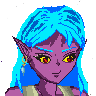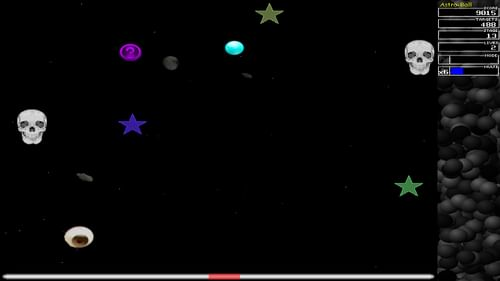-=+=- -=+=- -=+=- -=+=- -=+=- -=+=- -=+=- -=+=- -=+=- -=+=- -=+=- -=+=- -=+=- -=+=- -=+=- -=+=- -=+=- -=+=- -=+=- -=+=- -=+=- -=+=- -=+=- -=+=- -=+=- -=+=- -=+=- -=+=- -=+=- -=+=- (c) WidthPadding Industries 1987 0|605|0 -=+=- -=+=- -=+=- -=+=- -=+=- -=+=- -=+=- -=+=- -=+=- -=+=- -=+=- -=+=- -=+=- -=+=- -=+=- -=+=- -=+=- -=+=- -=+=- -=+=- -=+=- -=+=- -=+=- -=+=- -=+=- -=+=- -=+=- -=+=- -=+=- -=+=-

### Welcome to Socoder

Socoder is a community of coders, who sit in a quiet corner of the internet, chatting endlessly about random nonsense.
Occasionally we code, too.

If you'd like to join in with the chatter, feel free to register.

On the top left is a little cog which you can use to open the Settings sidebar, where you'll find the registration and login areas. Once you're logged in, this is also where most of the menu-based things will appear.
On the top right is a secondary sidebar button which can be used to reveal the most recent topics. You may dock either of these sidebars into place, using the provided links.
As well as these, there's a paintbrush on the top right to help you style the site using a variety of themes and other such settings.

Jayenkai7th October 2020Find the Disks, and rescue them from dampness.

spinal18th May 2020Monitor Menu is a simulation of an old CRT computer monitor settings menu

Tricky# Kitty - Console based Code Viewer

21st April 2020Kitty is a console based code viewer, with syntax highlight. The name is a pun on the Unix tool ''cat''.

The screenshot was demonstrated in PowerShell, but that's merely because I prefer PowerShell above cmd, as it should work in cmd as well*snip*

therevillsgames# Santa's Christmas Solitaire 2

20th December 2019Christmas + Solitaire = Santa's Christmas Solitaire 2

Tricky# Kthura - Map Editor

24th October 2019Let's show you my Kthura Map Editor

Kthura is a map system for 2D Map development, but here's the rub... It's not a tile sysem, but a object oriented map system. I originally designed it for an adventure game with a girl named Kthura as main*snip*

Tricky# Astrobounce

18th October 2019Astrobounce